• 3000 BCE

# Origin of Base-10 number system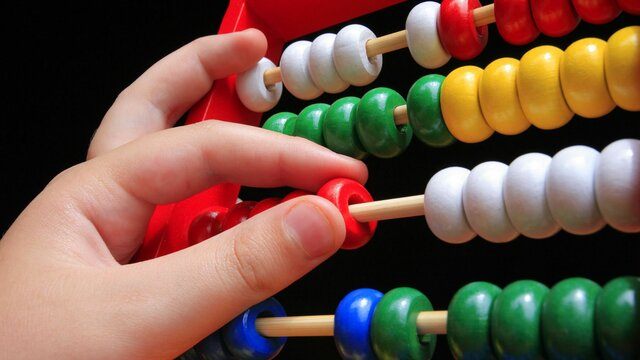Base-10 is used in most modern civilizations and was the most common system for ancient civilizations, most likely because humans have 10 fingers. Egyptian hieroglyphs dating back to 3000 B.C.
• 2000 BCE

# Arithmetic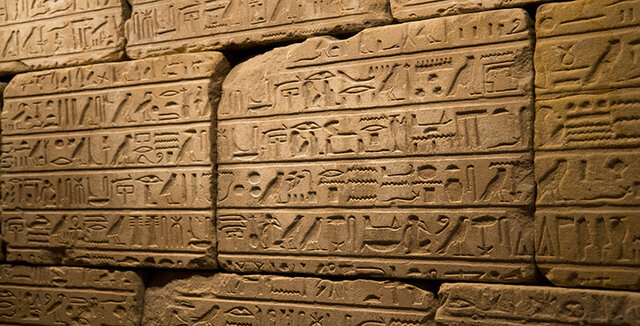The earliest written records indicate the Egyptians and Babylonians used all the elementary arithmetic operations as early as 2000 BC. These artifacts do not always reveal the specific process used for solving problems, but the characteristics of the particular numeral system strongly influence the complexity of the methods
• 1800 BCE

# History of Mathematics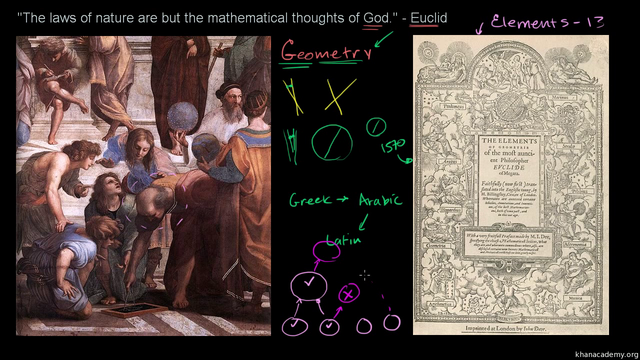Before the modern age and the worldwide spread of knowledge, written examples of new mathematical developments have come to light only in a few locales. From 3000 BC the Mesopotamian states of Sumer, Akkad and Assyria, followed closely by Ancient Egypt and the Levantine state of Ebla began using arithmetic, algebra and geometry for purposes of taxation, commerce, trade and also in the patterns in nature, the field of astronomy and to record time and formulate calendars.
• # NumeracyBefore the term number sense came into use, the word numeracy was coined in 1959 to denote those within the realm of mathematics who had a propensity to comprehend higher-level mathematical concepts.
• # Base Ten Blocks for elementary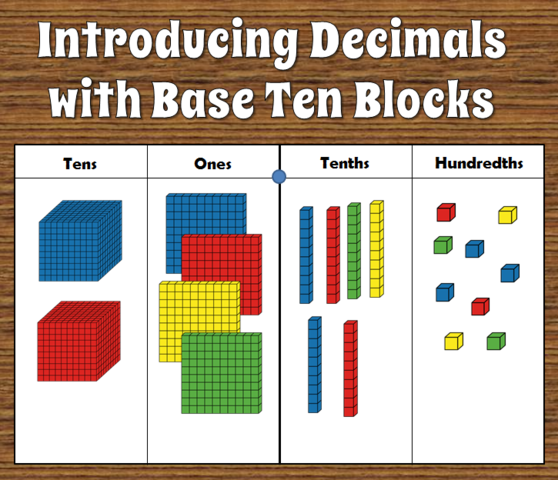Base ten blocks are popular in elementary school mathematics instruction, especially with topics that cause students struggle such as multiplication. They are frequently used in the classroom by teachers to model concepts, as well as by students to reinforce their own understanding of said concepts. Physically manipulating objects is an important technique used in learning basic mathematics principles, particularly at the early stages of cognitive development.
• # Warren McCulloch : "What is a number, that a man may know it, and a man, that he may know a number?"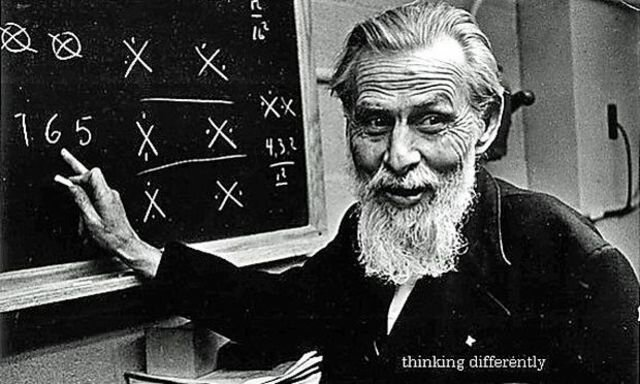The answer to this question changes with the understanding of mathematics. Mathematics educators have been researching how number sense ameliorates students' understanding of mathematics. Mathematics educators have embraced this shift toward a pedagogy that strives to merge intuition, formal notation, and conceptual understanding. Number sense helps students eschew the notion that mathematics is merely a collection of rules to memorize.
• # Innumeracy: Mathematical Illiteracy and Its Consequences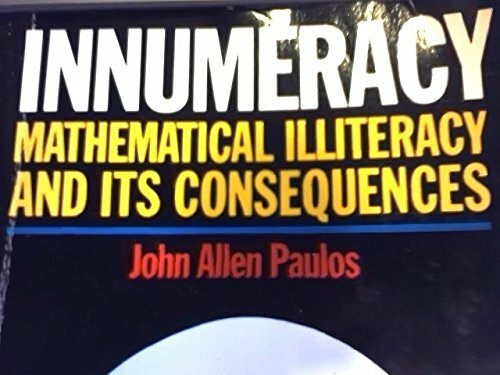A book by John Allen Paulos, Innumeracy: Mathematical Illiteracy and Its Consequences (1988), demonstrated the dangers of a population that lacks a basic understanding of mathematics and that views the subject as enigmatic due to poor education or psychological anxiety. Many of those involved with mathematics education felt that math pedagogy was in need of serious reform due to a superficial acquisition of knowledge based merely on a procedural understanding of mathematics.
• # The Number Sense: How the Mind Creates Mathematics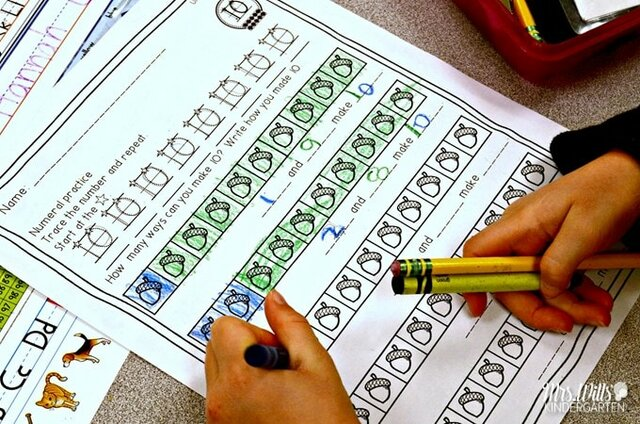The Number Sense: How the Mind Creates Mathematics, states, "Our number sense cannot be reduced to the formal definition provided by rules or axioms". In addition, James Greeno relates, "We recognize examples of number sense, even though we have no satisfactory definition that distinguishes its features"